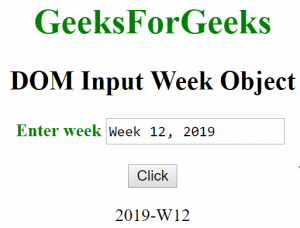# HTML | DOM Input Week Object

The Input Week object in HTML DOM represents an <input> element with type = “week” attribute. The element can be accessed by using getElementById() method.

Syntax:

`document.getElementById("id");`

where id is assigned to the <input> tag.

Property Values:

• list: It returns the reference of data list that contains the week field.
• form: It returns the reference of form that contains the week field.
• autocomplete: It is used to set or return the value of the autocomplete attribute of a week field.
• autofocus: It is used to set or return if the week field should automatically get focus when the page loads.
• defaultvalue: It is used set or return the default value of a week field.
• disabled: It is used to set or return whether a week field is disabled or not.
• max: It is used to set or return the value of the max attribute of a week field.
• min: It is used to set or return the value of the min attribute of a week field.
• name: It is used to set or return the value of the name attribute of a week field.
• readOnly: It is used to set or return whether the week field is read-only or not.
• required: It is used to set or return whether the week field must be filled out before submitting a form.
• step: It is used to set or return the value of the step attribute of the week field.
• type: It returns the type of form element the week field belongs.
• value: It is used set or return the value of the value attribute of a week field.

Methods

• stepDown(): It is used for decrementing the value of the week field by a specified number.
• stepUp(): It is used for incrementing the value of the week field by a specified number.
• select(): It is used to select the content of the Input week field.

Example 1: This example describes the getElementById() method to access the <input> element with type = “week” attribute.

## HTML

 ` ` `<``html``> ` `    ``<``head``> ` `        ``<``title``>` `            ``DOM Input Week Object ` `        ``` `    `` ` `    ``<``body``> ` `        ``<``center``> ` `            ``<``h1` `style` `= ``"color:green;"``> ` `                ``GeeksForGeeks ` `            `` ` `            `  `            ``<``h2``>DOM Input Week Object ` `                `  `            ``<``label` `for` `= ``"uname"` `style` `= ``"color:green"``>` `                ``<``b``>Enter week` `            ``` `            `  `            ``<``input` `type` `= ``"week"` `id` `= ``"gfg"` `                ``placeholder` `= ``"Enter week"``>` `            `  `            ``<``br``><``br``>` `                `  `            ``<``button` `type` `= ``"button"` `onclick` `= ``"geeks()"``> ` `                ``Click` `            `` ` `            `  `            ``<``p` `id` `= ``"GFG"``>`       `            `  `            ``<``script``> ` `                ``function geeks() { ` `                    ``var link = document.getElementById("gfg").value; ` `                    ``document.getElementById("GFG").innerHTML = link;` `                ``} ` `            `` ` `        ``` `    `` ` `                    `

Output:
Before Click on the button:After Click on the button:Example 2: This example describes the document.createElement() method to create <input> element with type = “week” attribute.

## HTML

 ` ` `<``html``> ` `    ``<``head``> ` `        ``<``title``>` `            ``DOM Input Week Object ` `        ``` `    `` ` `    `  `    ``<``body``> ` `        ``<``center``> ` `            ``<``h1` `style` `= ``"color:green;"``> ` `                ``GeeksForGeeks ` `            `` ` `            `  `            ``<``h2``>DOM Input Week Object ` `                `  `            ``<``label` `for` `= ``"uname"` `style` `= ``"color:green"``>` `                ``<``b``>Enter week` `            ``` `            `  `        ``<``p` `id` `= ``"GFG"``>`       `        `  `            ``<``button` `type` `= ``"button"` `onclick` `= ``"geeks()"``> ` `                ``Click` `            `` ` `            `  `            ``` `            ``<``script``> ` `                ``function geeks() {` `                    `  `                    ``/* Create input element  */` `                    ``var link = document.createElement("INPUT");` `                    `  `                    ``/* Set the type attribute */` `                    ``link.setAttribute("type", "week");` `                    `  `                    ``/* Append node value */` `                    ``document.getElementById("GFG").appendChild(link);` `                ``} ` `            `` ` `        ``` `    `` ` `                                          `

Output:
Before Click on the button:After Click on the button:Supported Browsers: